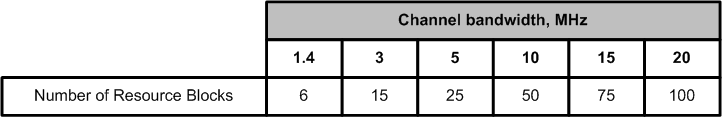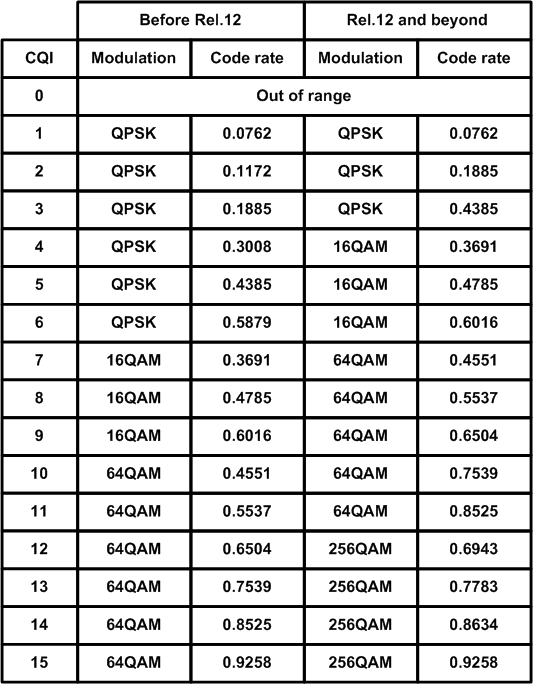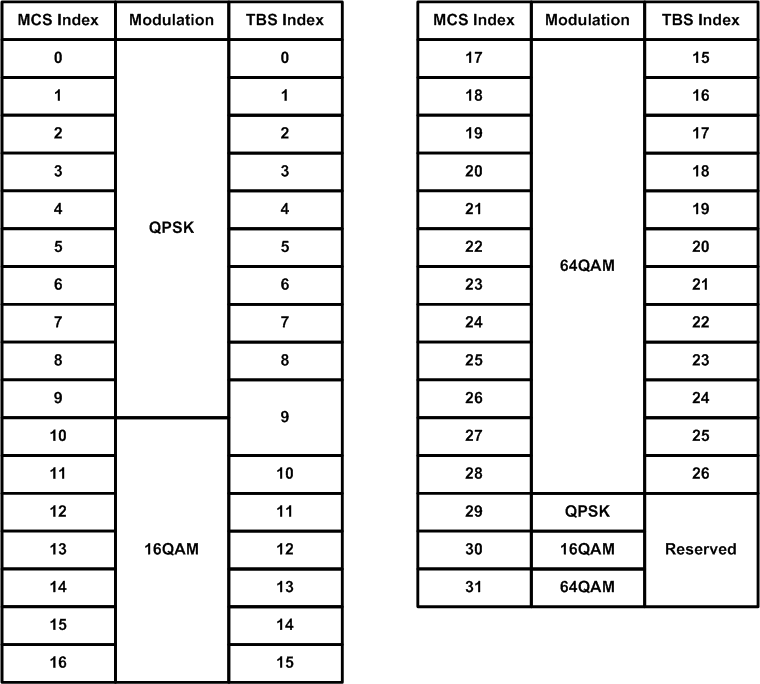﻿ How to calculate LTE throughput

How to calculate LTE throughput

LTE throughput calculator

Introduction

The post presents the simplest way how to calculate LTE throughput. The following calculations are made for user data bit rates on IP layer (including IP headers and LTE overhead, i.e. real user bit rate will be a bit lower). LTE throughput depends on the following parameters:
• Bandwidth
• Channel quality
Let's consider each of them in more details.
• Bandwidth. According to 3GPP specifications LTE channel bandwidth can be 1.4, 3, 5, 10, 15, 20 MHz. The wider bandwidth the higher throughput. All available spectrum is divided into Resource Blocks (RB). A table to map bandwidth on number of available RB is presented below.• Channel quality. Radio conditions impact user bit rates. The better radio conditions the higher throughput is available and vice versa. eNB (eNodeB, base station) selects Modulation and Coding Scheme (MCS) based on current radio conditions. The higher MCS the more bits can be transmitted per time unit. UE (User Equipment, mobile terminal) measures radio channel quality and sends CQI (Channel Quality Indicator) to eNB. eNB selects MCS based on the following table (it's presented for information only and will not be used for further throughput calculations).Also depending on radio conditions various multi-antenna techniques can be applied, e.g. MIMO 2x2 (Miltiple Input Multiple Output) or MIMO 4x4. These techniques help to increase throughput as well (almost in 2 or 4 times respectively).

• Network load. Available radio resources are divided among active subscribers. So the more subscribers are active and receive/transmit data the less resources are allocated to a given subscriber. It also depends on subscriber and connection (bearer in terms of LTE) priorities.

Throughput calculation

To calculate LTE throughput the following steps should be performed:
1. Define bandwidth. It can be 1.4, 3, 5, 10, 15 or 20 MHz. Then map it to a number of Resource Blocks using the table above.
2. Choose/define radio link quality. To calculate throughput we need to know MCS Index. MCS Index depends on CQI. However association between MCS Index and CQI is vendor specific. The following table can be used to choose MCS Index.where TBS means Transport Block Size. It's a number of bits which can be transmitted per 1 TTI (=1ms).
3. The last step is to take a look at Transport Block Size table to determine how many bits can be transmitted per 1 TTI and multiply it by 1000 to get bps.
LTE throughput calculator

Example

Let's assume there is a single UE, 20 MHz bandwidth and ideal radio conditions (to get peak throughput), then
Number of RBs = 100
MCS Index = 28 and TBS Index = 26

Based on that TBS = 75376 bits. Throughput = 75376 * 1000 = 75.376 Mbps. Assuming MIMO 2x2 we will get 75.376*2 = 150.752 Mbps. Please note there are additional TBS tables for MIMO cases defined in 3GPP specs.

You can share your feedback, questions and/or proposals sending an email to alexey.anisimov86[at]gmail.com

Alexey Anisimov, 2016